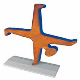# StepLineSeries - Last step

ChartViewTop achievementsRank 2
Maurizio asked on 16 Sep 2014, 03:11 PM
We are drawing a RadCartesianChart using a  StepLineSeries. So far so good.

How can we make the last step go until the end? Say our last data point is at (8;1) and the end of the x-axis is at 10. How can we make the ChartView draw a line from 8 to 10 to depict the last step (we would not like to add an additional point at (10;1).

See screenshot...

## 1 Answer, 1 is accepted

0Petar Marchev
Telerik teamanswered on 17 Sep 2014, 11:38 AM
Hello Peter,

In the ChartView controls set there are two different notions of axes - categorical and continuous. These are also explained in our online documentation.

The notion of the categorical axis (Categorical, DateTimeCategorical) is that each value is a category - a separate, discrete unit, that is un-measurable. Categories can be Bananas, Carrots, Bolts, DateTime, double, string and any other entity. A category is given its own portion of the axis - the distance between two ticks, its layout slot.

The notion of the continuous axes (Linear, Logarithmic, Polar, DateTimeContinuous) is that there are no discrete categories, but singularity values. Think of the linear axis. The value 6 has no layout slot, it only has a singularity along the linear axis, a point. The point series (also line series) describe this perfectly - points are located exactly where the value is. The line drawn between two values will denote exactly the first value and exactly the second value without prolonging the line. If the line was prolonged - this would add additional meaning to the line, meaning that it ends somewhere else and not where the second value is.

In the given set up, the snapshot you have attached, you have used a DateTimeContinuousAxis and the last point is drawn exactly where the last value is. If the line was prolonged - this would give additional meanint to the data, meaning that the last value did not just occur in a particular time, but it was preserved over time. If the the last point was indeed not a singularity event, but it continued some time, then it is only correct that an extra data item is added to denote the end of this period. So the answer to your question is to really add an extra item in your items collection to mark where this period ends.

Regards,
Petar Marchev
Telerik

Check out Telerik Analytics, the service which allows developers to discover app usage patterns, analyze user data, log exceptions, solve problems and profile application performance at run time. Watch the videos and start improving your app based on facts, not hunches.

Tags
ChartViewRank 2